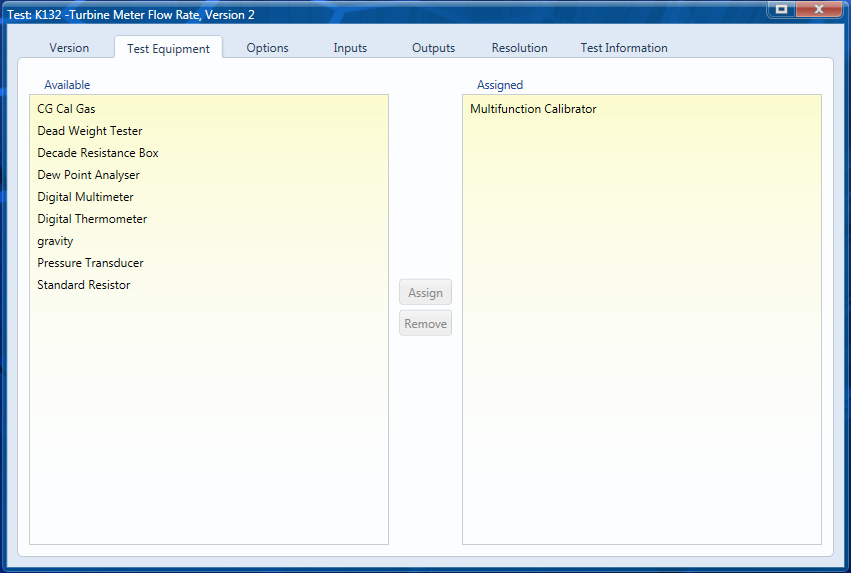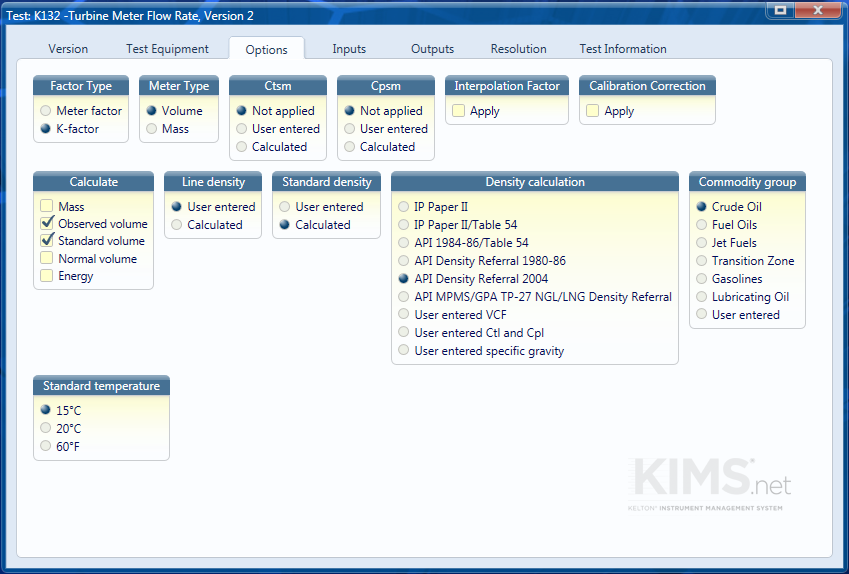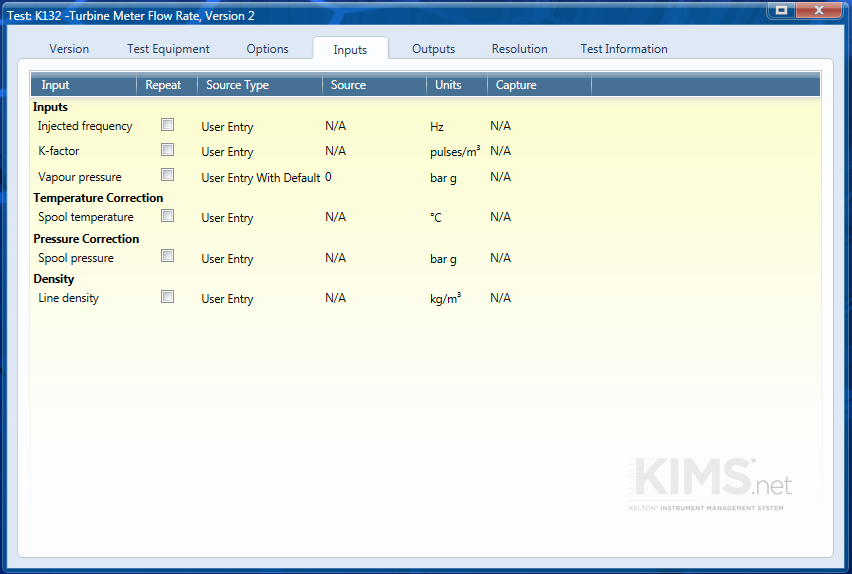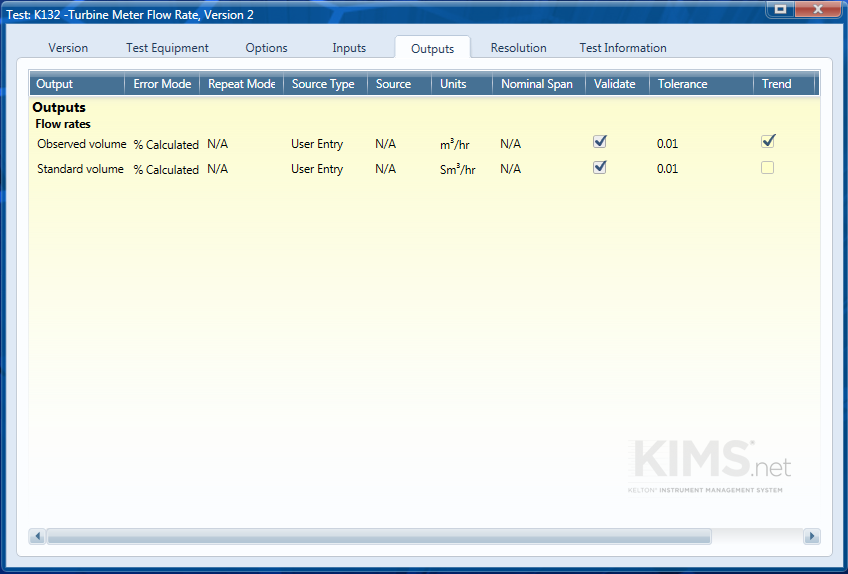# Turbine Meter Flow Rate Check

## Form

Form: K132 – Liquid Flow Rate – Pulse Output Meter

Form Reference: C050

Calculation Details

## Purpose

The purpose of this test is to check the pulse frequency from a meter is displaying the correct flow rate. This form will work for all meters where the output is in the form of a flow proportional frequency.

## Test Equipment

A multifunction calibrator is selected for the test equipment. Other options may include a signal generator and timer/counter.## Options

In this example we are checking a turbine meter where the output volume is proportional to the frequency. We are not correcting for Ctsm and Cpsm as the K-Factor is determined at the operating pressure and temperature. We are calculating both the volume at observed conditions and the standard volume. The standard density is calculated from the user entered line density using the 2004 API density Referral calculation for Cruse oil with a standard temperature of 15°C## Inputs

All inputs are to be entered at the time of the test. A default value of 0 bar g has been set for this instance as the vapour pressure is not determined.## Repeat Data

In this example there is nor repeat data; some users may repeat this test at different frequencies in which case the repeat box should be checked on the inputs tab.

## Outputs

The error mode will be percentage of calculated value

A typical tolerance for this check will be 0.01 % of calculated value.Back